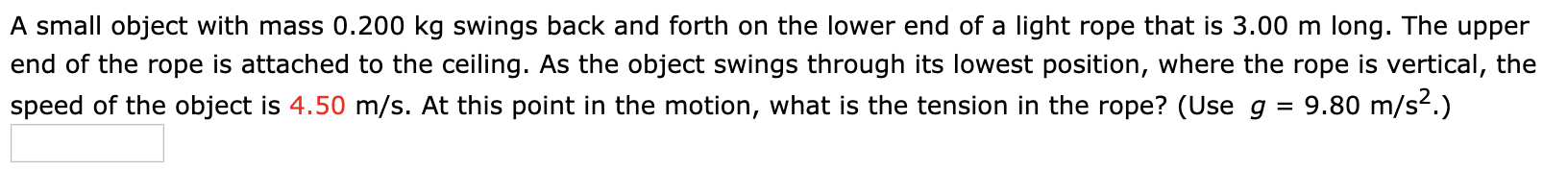# A small object with mass 0.200 kg swings back and forth on the lower end of a light rope that is 3.00 m long. The upper end of the rope is attached to the ceiling. As the object swings through its lowest position, where the rope is vertical, the speed of the object is 4.50 m/s. At this point in the motion, what is the tension in the rope? (Use g = 9.80 m/s2.)

Questionhelp_outlineImage TranscriptioncloseA small object with mass 0.200 kg swings back and forth on the lower end of a light rope that is 3.00 m long. The upper end of the rope is attached to the ceiling. As the object swings through its lowest position, where the rope is vertical, the speed of the object is 4.50 m/s. At this point in the motion, what is the tension in the rope? (Use g = 9.80 m/s2.) fullscreen

### Want to see the step-by-step answer?